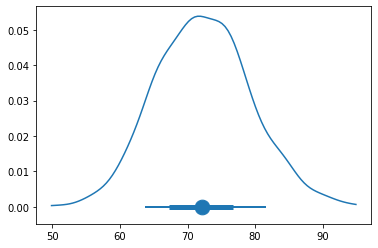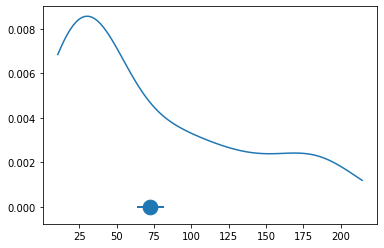## Konstantin Rebrov¶

### Lecture 10/23/2019¶

pseudocode for bootstrap method

for s in S:
generate random
if condition:
count += 1

count / sigma

Let's say for the sample mean, to get a confidence interval for a pop mean from the sample mean,

picks a lower bound for some percentile and pick unpper bound for some percentile in which we are 95%.

For the sample means most people construct confidence interals by +/- 2 standard errors.

IS symmetric confidence interval a useful quality? Edward: most popele would assume the CLT holds, it's symmetric done. But I would argue it's not the most useful because you're not guaranteed to have symmetric sampling distribution.

We are now starting official modeling in this class, likelihood mthod via minimization, and the bootstrap method. USing the simplified log likelihood for the normal distribution for the next 6 weeks of this class.

Quick review of the techniques.

Statistical modeling:

$N$ random variables

$X1, ..., X2 ~Normal(\mu, \sigma^{2})$

IF we don't need to estimate sigma, pretend all we;re estimated is the pop mean.

Entirley ignoring simga, after the deriv those pieces will fall away.

Simplified log likelihood template:

$-$ Sum of elements n=1 to $N$ of $(x_n - \mu)^{2}$ The $\mu$ will get changed

In :
import numpy as np
import bplot as bp
from scipy.stats import norm
from scipy.optimize import minimize
import pandas as pd


No negative 1, because we want to maximize, instead of minimize

In :
def ll_normal(mu, X):
d = X - mu
return np.sum(d * d)


Powers on computers are computationally expensive, se multiplication instead. Be efficient. I don't care whatever languag eyour in.

pandas allows us to access real data

github.com/roualdes/data is the repo for the data sets.

data frame is the standard name for a 2-D table matrix that holds your data in python. The difference between a 2-D array in python and a data frame, is a data frame has named columns. Similar to SQL tables.

73 rows, each row is a new book

Any time you want to download datasets, download the *.csv file , is a database. Read it into the python.

In :
df = pd.read_csv("https://raw.githubusercontent.com/roualdes/data/master/books.csv")


head prints the first 5 observations int eh data set, where each row is a book.

In :
df.head(8)  # 8 books first in the data set

Out:
isbn uclaNew amazNew
0 978-0803272620 27.67 27.95
1 978-0030119194 40.59 31.14
2 978-0300080643 31.68 32.00
3 978-0226206813 16.00 11.52
4 978-0892365999 18.95 14.21
5 978-0394723693 14.95 10.17
6 978-0822338437 24.70 20.06
7 978-0816646135 19.50 16.66

In python, function named dir(), I dont know what it stands for. MAkes a list of all the members and methods of the objects you call it on.

Konstantin: perhaps it is similar to ls in linux it is a listing of directories, so it is a listing of the members of objects.

In [ ]:
dir(df)

In [ ]:
help(pd.read_csv)


returns a padnas series object, but it is similar to columns. A series is wrapping an numpy array in a collumn.

In :
df['uclaNew']

Out:
0     27.67
1     40.59
2     31.68
3     16.00
4     18.95
...
68    48.46
69    39.55
70    29.65
71    23.76
72    27.70
Name: uclaNew, Length: 73, dtype: float64

You can perform vectorized math on columns in data frames.

In :
df['uclaNew'] + 2

Out:
0     29.67
1     42.59
2     33.68
3     18.00
4     20.95
...
68    50.46
69    41.55
70    31.65
71    25.76
72    29.70
Name: uclaNew, Length: 73, dtype: float64

Can you think of dataframes as holding multiple columns of variables? Yes. Are they stored in column major order? I believe they are actually stored in row manjoy order. numpy is row major. Other mathematical libraries use column major order.

In :
df.columns

Out:
Index(['isbn', 'uclaNew', 'amazNew'], dtype='object')

Take this new array of vars, and throw it into the bootstrap procedure.

In :
df['uclaNew']
df.shape

Out:
(73, 3)

Dataframes - 2-D arrays, shape is a 73 rows by 3 columns. Rows first, then columns. Returns a tuple.

In :
N = df.shape  # firs eelment of tuple
N

Out:
73

Resample from the original data $N$, the length of the original data $N$, with replacement replace=True

In :
R = 1001
mus = np.full(R, np.nan)
for r in range(R):
idx = np.random.choice(N, N, replace=True)
# N twice, is intentional

tmp = minimize(ll_normal, (50), args=(df['uclaNew'][idx]), method ="BFGS")

mus[r] = minimize(ll_normal, (50), args=(df['uclaNew'][idx]), method ="BFGS").x  # don't forget the x
# 50 is a reasonable starting guess for the price of a textbook, an optimization
# our array of random variables is a collumn of the dataframe, returns a numpy array
# mu can be -inf to +inf, no bounds on that param.
# BFGS can be used when there are no bounds.

tmp

Out:
      fun: 265131.8274740832
hess_inv: array([[0.00684931]])
jac: array([0.])
message: 'Optimization terminated successfully.'
nfev: 18
nit: 4
njev: 6
status: 0
success: True
x: array([74.43360056])

df['uclaNew'] is a numpy array df['uclaNew'][idx] returns the idx'th element

minimize returns a scipy.optimize.OptimizeResult, it is actually a dictionary.

x in that dictionalry contains the result of the best guess of the computation.

There are also other fields in the OptimizeResult

help(minimize)

In :
R = 1001
N = df.shape  # firs eelment of tuple
mus = np.full(R, np.nan)
for r in range(R):
idx = np.random.choice(N, N, replace=True)
mus[r] = minimize(ll_normal, (50), args=(df['uclaNew'][idx]), method ="BFGS").x  # don't forget the x

In :
bp.density(mus)
bp.percentile_h(mus, y=0)

Out:
<matplotlib.collections.PathCollection at 0x7f4f62f7a160>In :
mus.mean()

Out:
72.32311995065606

The true price of a textbook at the UCLA.

In :
bp.density(df['uclaNew'])
bp.percentile_h(mus, y=0)

Out:
<matplotlib.collections.PathCollection at 0x7f4f62811278>This curver, the data set of the original sample. Density plot tells us about the *individual books prices.

The confidence interval is tells us about the mean book price.

The confidence interval in this plot is coming form these sample statistics it is NOT a confidence interval for individual books prices. Do not confuse these two!

95% confident that the mean is aroudn that price.

Coudl we develop a function bootstrap() that will work with minimize()?

initval is a random guaess. It is a default parameter. Repeat the bootstrap with te new best guaess to avoid a local minimum.

In :
def min(data, initval = None):
if not initval:  # if no provided argument
initval = np.random.normal()
return minimize(ll_normal, (initval), args=(data), method ="BFGS").x  # don't forget the x
# [x] is also valid syntax

def bootstrap(data, R, fun):
N = data.size
thetas = np.full(R, np.nan)
for r in range(R):
idx = np.random.choice(N, N, replace = True)
thetas[r] = fun(data[idx])
return np.percentile(thetas, [25, 75])

R = 1001
bootstrap(df['uclaNew'], R, min)

Out:
array([67.26479132, 77.14575468])

HEre is a 95% confidence interval!

To repeat the analysis:

In :
R = 1001
bootstrap(df['amazNew'], R, min)

Out:
array([55.41272806, 63.60342724])

Amazon's book prices are cheaper than the University's bookstore.

min() is generally although a bad choice of a name, since it is common and it can be used in different contexts.